×#### Thank you for registering.

One of our academic counsellors will contact you within 1 working day.

Click to Chat

1800-1023-196

+91-120-4616500

CART 0

• 0

MY CART (5)

Use Coupon: CART20 and get 20% off on all online Study Material

ITEM
DETAILS
MRP
DISCOUNT
FINAL PRICE
Total Price: Rs.

There are no items in this cart.
Continue Shopping• Complete Physics Course - Class 11
• OFFERED PRICE: Rs. 2,968
• View Details

```Effect of Dielectric on Capacitance

Table of Content

What is Dielectric?

What is Dielectric Constant?

Effect of Dielectric on Capacitance

How dielectric increase the capacitance of capacitor?

Dielectric Polarisation

Inserting dielectric in Capacitor

Effect of dielectric on plates when voltage or charge is held constant

What does a dielectric do to a capacitor?

Capacitance of Parallel Plate Capacitor

Capacitance of Parallel Plate Capacitor when dielectric slab is placed

Capacitance in the presence of Dielectric

What is Dielectric?

Dielectrics are non-conducting substanceswhich are the insulating materials and are bad conductor of electric current.Dielectric materials can be made to hold an electrostatic charge while dissipating minimal energy in the form of heat. Examples of Dielectric are Mica, Plastics, Glass, Porcelain and Various Metal Oxides and even dry air is also example of dielectric.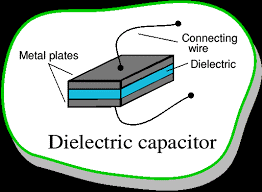Figure 1.1 Dielectric capacitor

What is Dielectric Constant?

In parallel plate capacitor, when dielectric slab is placed between the two plates then the ratio of the applied electric field strength to the strength of the reduced value of electric field capacitor is called dielectric constant that is:

K = Eo/E

K is dielectric constant

E0 is greater then or equal to E

Where Eo is dielectric

And  E is net field

The larger the dielectric constant, the more charge can be stored. Completely filling the space between capacitor plates with a dielectric increases the capacitance by a factor of the dielectric constant:

C = KCo, where Co is the capacitance with no dielectric between the plates.

Effect of Dielectric on Capacitance

Dielectrics are usually placed between the two plates of parallel plate capacitors. They can fully occupy the region between the plates or can partially occupy.

When the dielectric is placed between the two plates of parallel plate capacitor, it is polarized by the electric field present. The surface charge densities are considered as σp and – σp.

When the dielectric is fully placed between the two plates of capacitor then it’s dielectric constant increases from it’s vacuum value.Figure 1.2 Dielectric placed between two plates

Electric field inside a capacitor is given by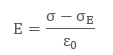So,And,Ɛ is the permittivity of the substance.

Potential difference between the plates is given byFor linear dielectrics,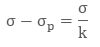Where k is dielectric constant of the substance

K = 1 else ,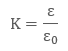How dielectric increase the capacitance of capacitor?

The electric field between the plates of parallel plate capacitor is directly proportional to capacitance C of the capacitor. The strength of electric field is reduced due to presence of dielectric and if the total charge on the plates is kept constant then the potential difference is reduced across the capacitor plates.

In this way dielectric increases the capacitance of capacitor.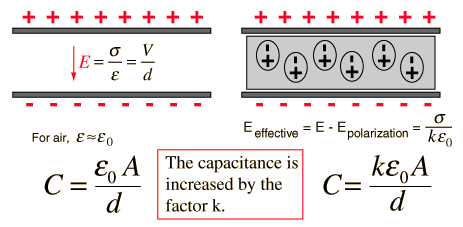Figure 1.3  Parallel Plate capacitor with dielectric

Proof

Consider the following diagramFig 1.4 dielectric between two capacitors

Dielectric Polarisation

When an external electric field is applied to a dielectric material, its behavior can be determined and is known as Dielectric Polarization that can be understood by the displacement of charges(positive and negative) when an electric field is applied

The main task of the dielectric polarization is to relate macroscopic properties to microscopic properties. Where macroscopic property can be dielectric constant to polarizability

Polarization occurs through the action of an electric field or other external factors, such as mechanical stress in the case of piezoelectric crystals(piezoelectric crystals are those solid materials which accumulates electric charge within them). Dielectric polarization can also arise spontaneously in pyroelectric crystals, particularly in ferroelectrics (Ferroelectricity is a property of certain materials that have a spontaneous electric polarization that can be reversed by the application of an external electric field).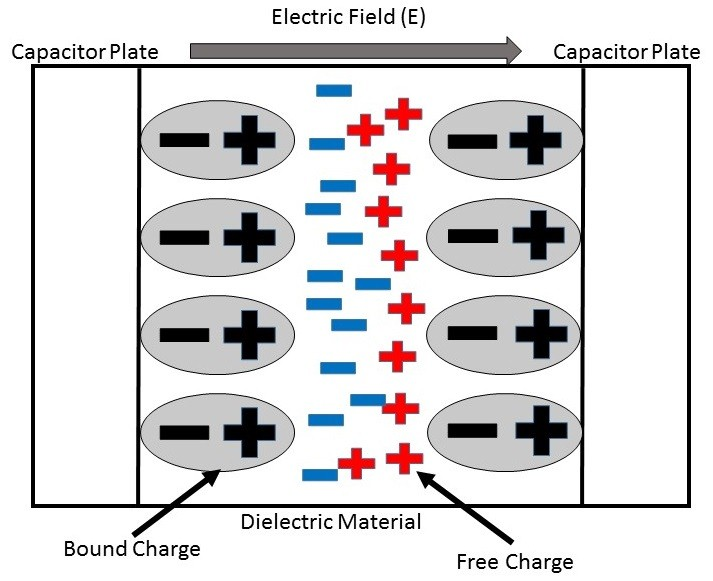1.5 Dielectric Polarisation

Inserting dielectric in Capacitor

When a dielectric material is introduced between the plates And when a dielectric material is placed between the plates of parallel plate capacitor then due to polarization of charges on either side of the dielectric, it produces an electric field of its own which acts in a direction opposite to that of the field due to the source.

This makes the net electric flux zero. This effect results in capacitance.

Effect of dielectric on plates when voltage or charge is held constant

Inserting a dielectric between the plates of capacitor while either the voltage or charge is held constant has the same effect that is the ratio of charge to voltage increases.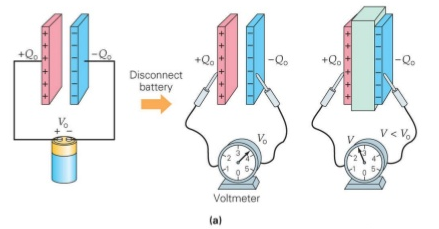Figure 1.6 Effect of dielectric on plates when voltage or the charge is held constant

What does a dielectric do to a capacitor?

Dielectrics are often called Insulators when the insulator are introduced between the two plates of capacitor.

The side of the insulator closest to the positive plate will be negative, while the side closest to the negative plate will be positive.

The net field inside the capacitor and the potential difference across the capacitor is decreased when the electric field from the charge of capacitor plates are cancelled by the electric field from the polarized dielectric.More charge is needed by the capacitor to return to its original potential difference.

The net effect of the dielectric is to increase the amount of charge a capacitor can store for a given potential difference. The whole point of using a capacitor is to store charge, so coming up with a way to store more charge for the same amount of effort is a good thing.

Adding dielectric to a capacitor increases the capacitance by a factor of K and,

Charge on the capacitor will increase when the voltage is held constant and

If the capacitor is disconnected thatis the charge is held constant the potential across the capacitor will decrease.

Capacitance of Parallel Plate Capacitor

The capacitance of parallel plate capacitor depends upon:

The distance d between two plates

The area A of medium between the plates

According to the gauss law, the electric field can be written asSince we know that the capacitance is defined as V = Q/C, so capacitance can be rewritten as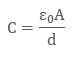When the plates areplaced very close and the area of plates are large we get the maximum capacitance.

Capacitance of Parallel Plate Capacitor when dielectric slab is placed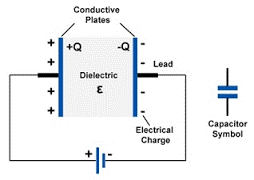Fig 1.7 dielectric placed between two electrodes

On the two plates, the microscopic dipole moment of the material will shield the charges. Thus, will alter the effect of dielectric material which is inserted between the two plates Materials have a permeability which is given by the relative permeability K

The capacitance is thus given by: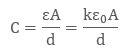Capacitance of a parallel plate capacitor can be increased by introducing dielectric between the plates as the dielectric have permeability k, which is greater than 1. K is also sometimes known as Dielectric Constant.

Capacitance in the presence of Dielectric

1. When dielectric is completely filled between the plates

When the area between the two plates is filled completely with dielectric then of relative permeability er,

C0 = C x er

The presence of dielectric will reduce the electric intensity by er times

Thus the capacitance is increased by er times.

2. When dielectric is partially filled between the plates

When in parallel plate capacitor the area between the who plates are partially filled with air and partially with other substance its Capacitance can be calculate.

Let there exist a parallel plate capacitor in which medium between the parallel plates is mainly the air and partially other substance.

Effect of Dielectrics on Capacitance
```### Course Features

• 101 Video Lectures
• Revision Notes
• Previous Year Papers
• Mind Map
• Study Planner
• NCERT Solutions
• Discussion Forum
• Test paper with Video Solution